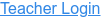### Topics

• Multiplication
• Decimals
• Area Model
• Factors
• Partial Products

### Description

Build rectangles of various sizes and relate multiplication to area. Partition a rectangle into smaller areas and discover new strategies for multiplying decimals!

### Sample Learning Goals

• Recognize that area represents the product of two numbers and is additive.
• Develop and justify a strategy that uses the area model to simplify a decimal multiplication problem.
• Devise a strategy to determine the product of two rational numbers (or an integer and a rational number) by representing the product as an area or the sum of areas.
• Use an area model to understand why multiplying a given number by a decimal less than 1 results in a product smaller than the given number.
• Interpret the product of two rational numbers using base-ten tiles
Version 1.0.0